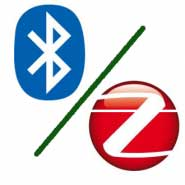# Difference between zigbee and Bluetooth

There are the difference between zigbee and Bluetooth are given below,

Zigbee  Bluetooth
The DSSS (Direct sequence spread spectrum) modulation techniques are used. The FHSS (Frequency hopping spread spectrum) modulation techniques are used.
It has less power consumption. It is high power consumption.
The communications range is up to 70 meters. The communications range is 1 or 100 meters, depending on radio class.
Its battery is not rechargeable because batteries will last for up to 10 years. In its battery frequent charging is required.
The protocol stack size is 28 K bytes. The protocol size is 250 K bytes.
The maximum number of cell nodes is more than 65000. The maximum number of cell nodes is up to 8.
The IEEE standard is 802.15.4. The IEEE standard is 802.15.1.
The maximum network speed is 250 bit per second. The maximum network speed is 1 M bit per second.
The typical network join time is 30 milliseconds. The typical network join time is 3 seconds.
The frequency band is 2.4 GHz, 868 MHz and 915 MHz. The frequency band is 2.4 GHz.
The Star, tree, cluster tree, and mesh topology are used. Tree topology is used.